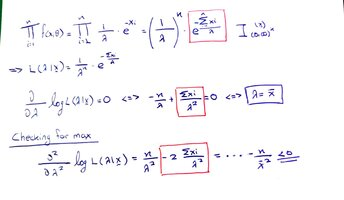# Finding a Maximum Likelihood Estimator (X=(X1,X2,...,Xn) random sample ~ E(λ))

#### mihalaras

##### New member
Description
We have X=(X1,X2,...,Xn) random sample ~ E(λ)

Finding a Maximum Likelihood Estimator:My Questions:
I have marked with red color the parts that I'm facing an issue to understand
1) I can't understand why Π {e^(-xi)} has a "/n" when we turn it into a Σ. Should it not be just "e^Σ(-xi)" ?
2) What is the logic behind finding the derivative of the first red marked term and turning it into the second red marked term? What rule do we follow to do this?
3) Basically the same as number 2, as we take the second derivative and we have the third red marked term. Again, if there is a rule that explains this, it would be very helpful.

#### blamocur

##### Senior Member
Regarding 1): I didn't find any "/n", but have "[imath]/\lambda[/imath]" comes from the definition of the exponential distribution. BTW, the previous term in that equality is incorrect, which might be the cause of your confusion.

•stapel and mihalaras

#### blamocur

##### Senior Member
2) What is the logic behind finding the derivative of the first red marked term and turning it into the second red marked term? What rule do we follow to do this?
The logic of find the derivative is to help in maximizing the likelihood. Since [imath]\log[/imath] is a monotonic function its maximum is achieved for the same value of [imath]\lambda[/imath], but the expression is simpler.

•stapel

#### blamocur

##### Senior Member
3) Basically the same as number 2, as we take the second derivative and we have the third red marked term. Again, if there is a rule that explains this, it would be very helpful.
Once you find the point with zero first derivative you use the second derivative to make sure that the critical point corresponds to the maximum (as opposed to minimum or inflection point).

P.S. Have you learned any calculus?

•stapel

#### mihalaras

##### New member
The logic of find the derivative is to help in maximizing the likelihood. Since [imath]\log[/imath] is a monotonic function its maximum is achieved for the same value of [imath]\lambda[/imath], but the expression is simpler.
Thanks for your answers. You are right about 1), however there is a slight misunderstanding on what I asked on numbers 2) and 3). I didn't explain it correctly at the beginning, so let me try to rephrase.

You explained me the theoretical reason on the steps that we follow, which I can understand.

What I struggled to understand was the practical finding of the derivative e^(Σ(-xi)/λ) which turns out to be Σxi/λ^2 . What is the formula that we follow in order to find this one out?

#### BigBeachBanana

##### Senior Member
Thanks for your answers. You are right about 1), however there is a slight misunderstanding on what I asked on numbers 2) and 3). I didn't explain it correctly at the beginning, so let me try to rephrase.

You explained me the theoretical reason on the steps that we follow, which I can understand.

What I struggled to understand was the practical finding of the derivative e^(Σ(-xi)/λ) which turns out to be Σxi/λ^2 . What is the formula that we follow in order to find this one out?

• That's the incorrect density function for the exponential function (pointed out above)
• The maximum likelihood is incorrect. It should be [imath]\lambda = \dfrac{1}{\bar{x}}[/imath]
• The second derivative should be divided by [imath]\lambda^3[/imath] not [imath]\lambda^2[/imath]

Last edited:
•stapel and mihalaras

#### blamocur

##### Senior Member
What I struggled to understand was the practical finding of the derivative e^(Σ(-xi)/λ) which turns out to be Σxi/λ^2 .
It is not the derivative of [imath]e^{\sum x_i / \lambda}[/imath] but of log of [imath]e^{\sum x_i / \lambda}[/imath]. Does it make sense now?

•stapel and mihalaras

#### mihalaras

##### New member

• That's the incorrect density function for the exponential function (pointed out above)
• The maximum likelihood is incorrect. It should be [imath]\lambda = \dfrac{1}{\bar{x}}[/imath]
• The second derivative should be divided by [imath]\lambda^3[/imath] not [imath]\lambda^2[/imath]
Thank you a lot for the corrections!

It is actually from notes of a classmate of mine, so it's possible he copied something wrong.

#### mihalaras

##### New member
It is not the derivative of [imath]e^{\sum x_i / \lambda}[/imath] but of log of [imath]e^{\sum x_i / \lambda}[/imath]. Does it make sense now
Oh yes, you are right. Thank you a lot for your time.

#### BigBeachBanana

##### Senior Member
Thank you a lot for the corrections!

It is actually from notes of a classmate of mine, so it's possible he copied something wrong.

•mihalaras

#### khansaheb

##### Full Member
Description
We have X=(X1,X2,...,Xn) random sample ~ E(λ)

Finding a Maximum Likelihood Estimator:

View attachment 36417
My Questions:
I have marked with red color the parts that I'm facing an issue to understand
1) I can't understand why Π {e^(-xi)} has a "/n" when we turn it into a Σ. Should it not be just "e^Σ(-xi)" ?
2) What is the logic behind finding the derivative of the first red marked term and turning it into the second red marked term? What rule do we follow to do this?
3) Basically the same as number 2, as we take the second derivative and we have the third red marked term. Again, if there is a rule that explains this, it would be very helpful.